# Electrical resistance

Electrical resistance definition and calculations.

## Resistance definition

Resistance is an electrical quantity that measures how the device or material reduces the electric current flow through it.

The resistance is measured in units of ohms (Ω).

If we make an analogy to water flow in pipes, the resistance is bigger when the pipe is thinner, so the water flow is decreased.

## Resistance calculation

The resistance of a conductor is resistivity of the conductor's material times the conductor's length divided by the conductor's cross sectional area.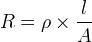R is the resistance in ohms (Ω).

ρ is the resistivity in ohms-meter (Ω×m)

l is the length of the conductor in meter (m)

A is the cross sectional area of the conductor in square meters (m2)

It is easy to understand this formula with water pipes analogy:

• when the pipe is longer, the length is bigger and the resistance will increase.
• when the pipe is wider, the cross sectional area is bigger and the resistance will decrease.

### Resistance calculation with ohm's law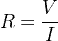R is the resistance of the resistor in ohms (Ω).

V is the voltage drop on the resistor in volts (V).

I is the current of the resistor in amperes (A).

### Temperature effects of resistance

The resistance of a resistor increases when temperature of the resistor increases.

R2 = R1 × ( 1 + α(T2 - T1) )

R2 is the resistance at temperature T2 in ohms (Ω).

R1 is the resistance at temperature T1 in ohms (Ω).

α is the temperature coefficient.

### Resistance of resistors in series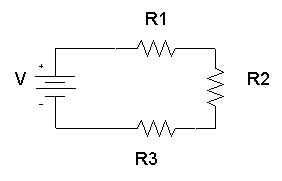The total equivalent resistance of resistors in series is the sum of the resistance values:

RTotal = R1+ R2+ R3+...

### Resistance of resistors in parallel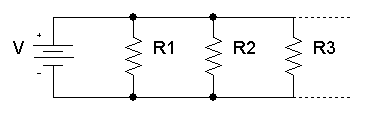The total equivalent resistance of resistors in parallel is given by: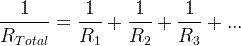### Measuring electrical resistance

Electrical resistance is measured with ohmmeter instrument.

In order to measure the resistance of a resistor or a circuit, the circuit should have the power supply turned off.

The ohmmeter should be connected to the two ends of the circuit so the resistance can be read.

### Superconductivity

Superconductivity is the drop of resistance to zero at very low temperatures near 0ºK.

Currently, we have around 5612 calculators, conversion tables and usefull online tools and software features for students, teaching and teachers, designers and simply for everyone.